Test: Optimal Power System Operation of Power Systems

# Test: Optimal Power System Operation of Power Systems - Electrical Engineering (EE)

Test Description

## 10 Questions MCQ Test GATE Electrical Engineering (EE) 2023 Mock Test Series - Test: Optimal Power System Operation of Power Systems

Test: Optimal Power System Operation of Power Systems for Electrical Engineering (EE) 2023 is part of GATE Electrical Engineering (EE) 2023 Mock Test Series preparation. The Test: Optimal Power System Operation of Power Systems questions and answers have been prepared according to the Electrical Engineering (EE) exam syllabus.The Test: Optimal Power System Operation of Power Systems MCQs are made for Electrical Engineering (EE) 2023 Exam. Find important definitions, questions, notes, meanings, examples, exercises, MCQs and online tests for Test: Optimal Power System Operation of Power Systems below.
Solutions of Test: Optimal Power System Operation of Power Systems questions in English are available as part of our GATE Electrical Engineering (EE) 2023 Mock Test Series for Electrical Engineering (EE) & Test: Optimal Power System Operation of Power Systems solutions in Hindi for GATE Electrical Engineering (EE) 2023 Mock Test Series course. Download more important topics, notes, lectures and mock test series for Electrical Engineering (EE) Exam by signing up for free. Attempt Test: Optimal Power System Operation of Power Systems | 10 questions in 30 minutes | Mock test for Electrical Engineering (EE) preparation | Free important questions MCQ to study GATE Electrical Engineering (EE) 2023 Mock Test Series for Electrical Engineering (EE) Exam | Download free PDF with solutions
 1 Crore+ students have signed up on EduRev. Have you?
Test: Optimal Power System Operation of Power Systems - Question 1

### If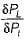for a plant i with output P1 and loss in the system being PL increases, the penalty factor of the system

Detailed Solution for Test: Optimal Power System Operation of Power Systems - Question 1

Penalty factor of the system is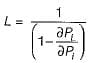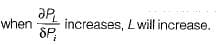Test: Optimal Power System Operation of Power Systems - Question 2

### The incremental fuel costs of two plants are given by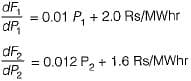For economic schedule of a load of 180 MW

Detailed Solution for Test: Optimal Power System Operation of Power Systems - Question 2

For economic scheduling of plants, we have: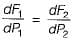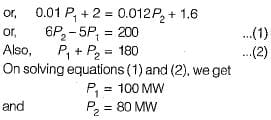Test: Optimal Power System Operation of Power Systems - Question 3

### A generator is supplying a load. An incremental change in load of 4 MW requires generation to be increased by 6 MW. The cost at plant bus is ₹ 30/MWhr. The incremental cost at the receiving end is

Detailed Solution for Test: Optimal Power System Operation of Power Systems - Question 3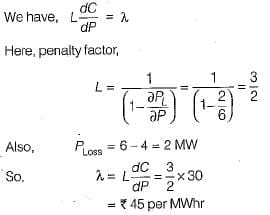Test: Optimal Power System Operation of Power Systems - Question 4

The current distribution factors for the power system network shown below are given by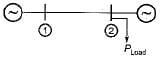Detailed Solution for Test: Optimal Power System Operation of Power Systems - Question 4

Since the load is at plant 2 therefore,
Na2 = 0 and Na1 = 1

Test: Optimal Power System Operation of Power Systems - Question 5

The incremental transmission loss of a plant can’t be a

Detailed Solution for Test: Optimal Power System Operation of Power Systems - Question 5

The incremental transmission loss of a plant can be positive, a non-zero value but, can’t be zero.

Test: Optimal Power System Operation of Power Systems - Question 6

When generating units are loaded to equal incremental costs, it results in

Detailed Solution for Test: Optimal Power System Operation of Power Systems - Question 6

To minimize the total fuel cost, the generating units must operate at equal incremental cost of production.

Test: Optimal Power System Operation of Power Systems - Question 7

Unit of heat rate curve is

Detailed Solution for Test: Optimal Power System Operation of Power Systems - Question 7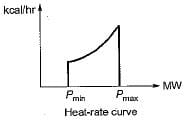Test: Optimal Power System Operation of Power Systems - Question 8

Unit of λ is

Detailed Solution for Test: Optimal Power System Operation of Power Systems - Question 8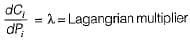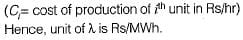Test: Optimal Power System Operation of Power Systems - Question 9

Cost curves are expressed as:

Test: Optimal Power System Operation of Power Systems - Question 10

The optimization problem is

## GATE Electrical Engineering (EE) 2023 Mock Test Series

22 docs|284 tests
Information about Test: Optimal Power System Operation of Power Systems Page
In this test you can find the Exam questions for Test: Optimal Power System Operation of Power Systems solved & explained in the simplest way possible. Besides giving Questions and answers for Test: Optimal Power System Operation of Power Systems, EduRev gives you an ample number of Online tests for practice

## GATE Electrical Engineering (EE) 2023 Mock Test Series

22 docs|284 tests(Scan QR code)